Multiprocess
Multilevel ModelingaML

### Likelihood and Predictions of Multinomial Probit Model

This note describes how to calculate the likelihood of a multinomial probit. By trivial extension, it permits calculating the predicted probabilities of an estimated multinomial probit model.

Consider a binomial probit with potential outcomes Y∈{0,1,2}. The discussion below readily generalizes to higher-order multinomial probits. Associated with each outcome is a so-called value function. We arbitrarily select Y=0 as the omitted category, i.e., with zero value function: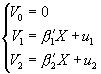where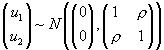. The outcome corresponds to whichever value function is greatest: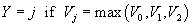.
The likelihood is: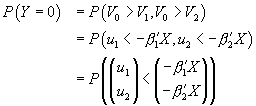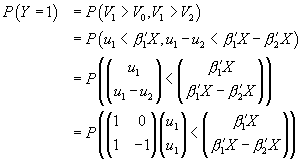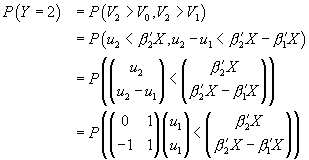All three probabilities involve a cumulative bivariate normal probability. The first is trivial: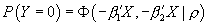. For the others, we first need to determine the bivariate covariance matrices. The covariance matrix for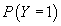is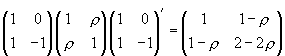and thus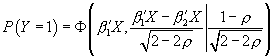. The last covariance matrix is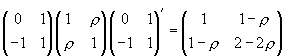and thus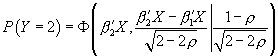.

Internally, aML Version 2.0 follows a generalized version of the above discussion: it converts every n-nomial probit outcome into n correlated probit outcomes.

The likelihood and predicted probabilities may readily be estimated using any statistical package that supports the bivariate cumulative normal probability function. We illustrate the implementation in Stata with ado file binphat.ado (binomial probit hat). It takes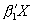,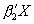, and ρ as inputs and generates three probability variables corresponding to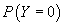,, and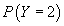.